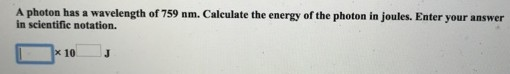# Problem: A photon has a wavelength of 759 nm. Calculate the energy of the photon in joules. Enter your answer in scientific notation.

###### FREE Expert Solution
99% (234 ratings)###### Problem Details

A photon has a wavelength of 759 nm. Calculate the energy of the photon in joules. Enter your answer in scientific notation.Frequently Asked Questions

What scientific concept do you need to know in order to solve this problem?

Our tutors have indicated that to solve this problem you will need to apply the The Energy of Light concept. You can view video lessons to learn The Energy of Light. Or if you need more The Energy of Light practice, you can also practice The Energy of Light practice problems.

What professor is this problem relevant for?

Based on our data, we think this problem is relevant for Professor Zhang's class at WSU.